# Graphing Relations

There are two ways to think about drawing a picture of a relation on a set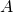.

### if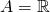We can draw relations on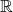, because they are subsets of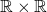-- i.e., subsets of the plane. Actually you've been drawing these pictures since way back.

For example, let's consider the relation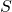given by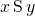if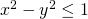. The picture looks like: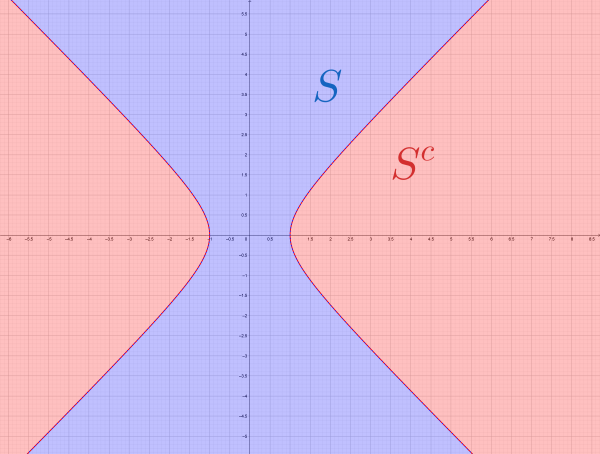Here I've putin blue, and its complement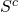in red.

What does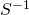look like?means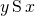, i.e.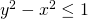. Here's that, in green.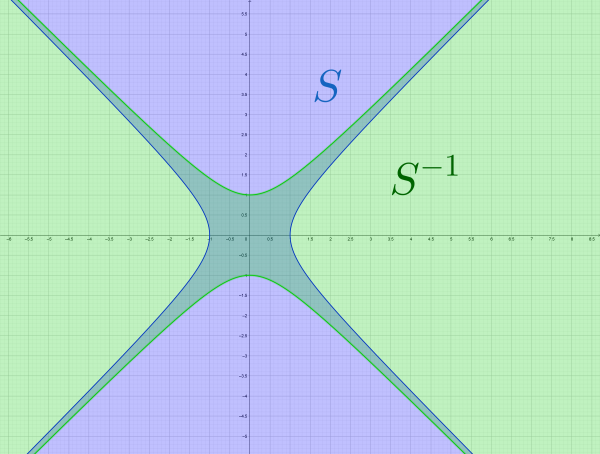Notice thatandare general rather different things (that's as we saw before).

To getfrom, we swap the roles of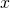and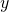. visually, this looks like reflection across the line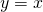. Here's another example, with the linedraw on explicitly: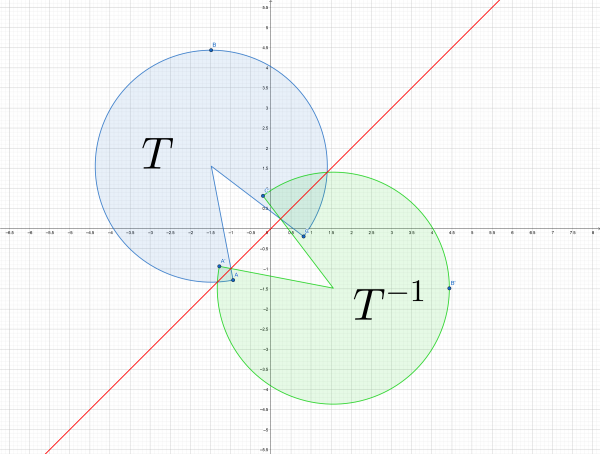We can use such a visual depiction to compute the domain and range of a relation: the domain is the "horizontal shadow" and the range is the "vertical shadow: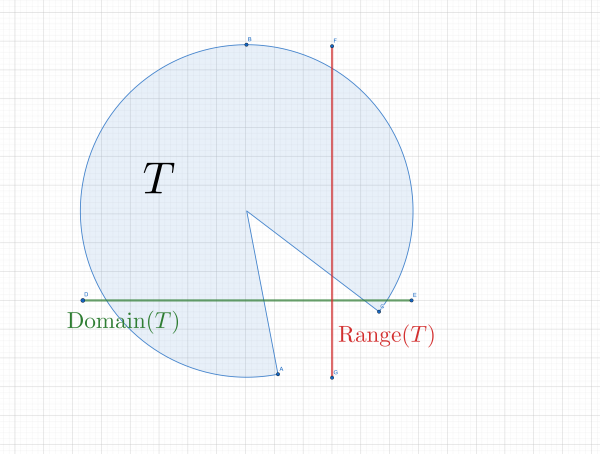We can also use such a depiction to check some properties of relations. For example: for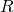to be reflexive onmeans every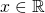has. That is, every pair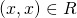. All of the pairs of the form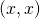constitute the diagonal line. Thus we can check reflexivity by asking: does the relation contain the diagonal line?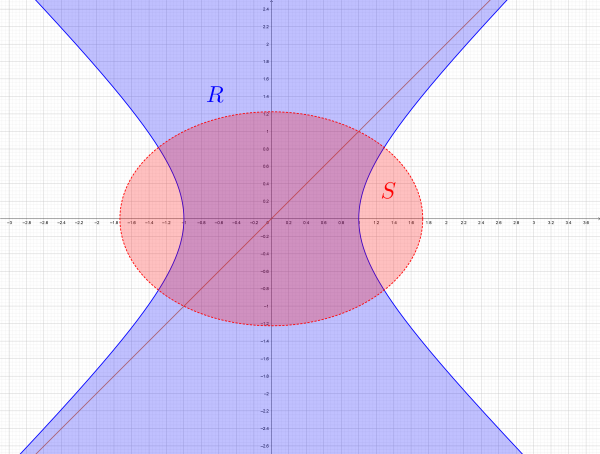Here the relationis reflexive --- it contains the line--- and the relationis not.

We can also check symmetry. Forto be symmetric means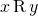guarantees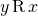. That is, if we reflectacross the line, we should land in inagain. That is,being symmetric meansis symmetric about the line. Neithernorabove is symmetric.

Transitivity is a bit more fun to check. Transitivity is relevant to pairs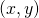and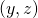both in. Here we'll check whether, given byif, is transitive. We have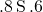and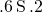, so we check to see if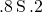. Here,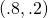is indicated in red --- it's in. But we need this to hold for every such pair of points in.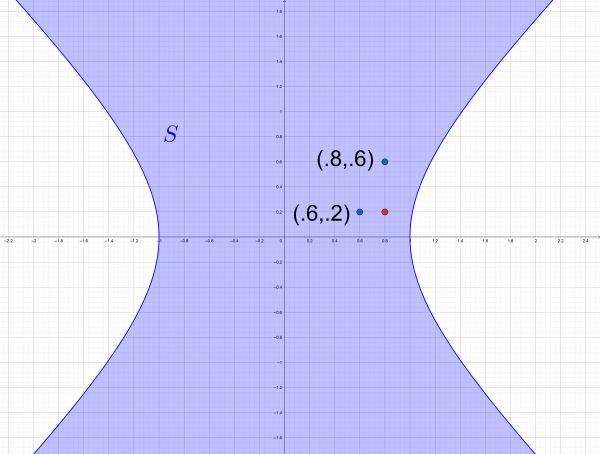Unfortunately, it does not: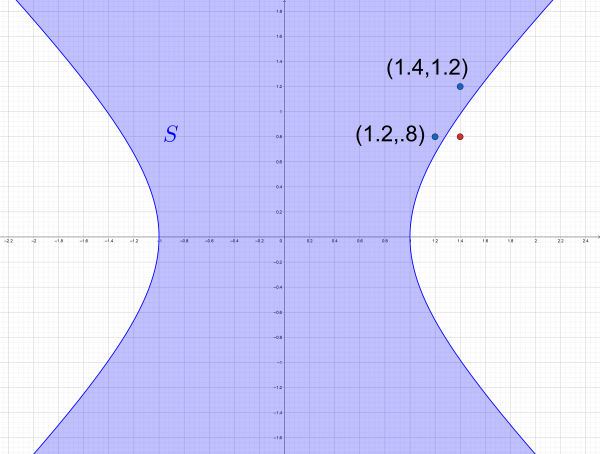It's a bit hard to see visually just what transitivity means, but you can see that it's got something to do with right triangles.

### ifis a finite set

Ifis a finite set, we can draw what's called a directed graph, or digraph, of the relation. A directed graph is a collection of vertices, which we draw as points, and directed edges, which we draw as arrows between the points. For example, let's take the set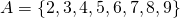and the relation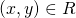if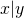.

The digraph corresponding to this relation is draw like this: we know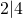,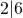, and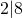. So we draw three arrows starting from 2. We also know that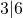and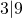, so we draw those: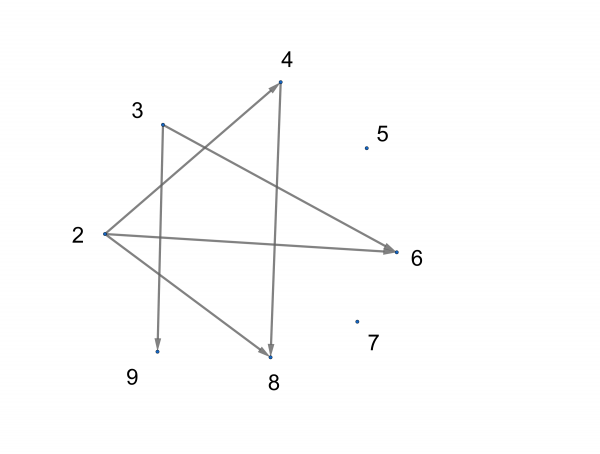But that's not all. We also know that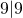, for example. So we need to add arrows that start and end at each vertex: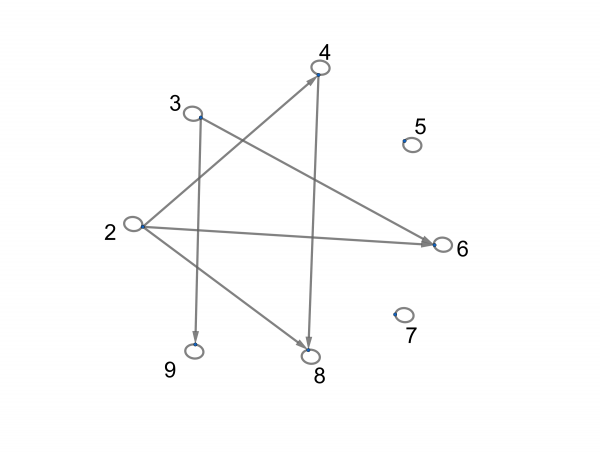Observe that divides, as a relation on this set, is transitive: whenever we haveand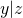, we automatically have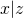. We can recognize this in the digraph by checking that, whenever there are two arrows connected head to tail, the third leg of that triangle is present: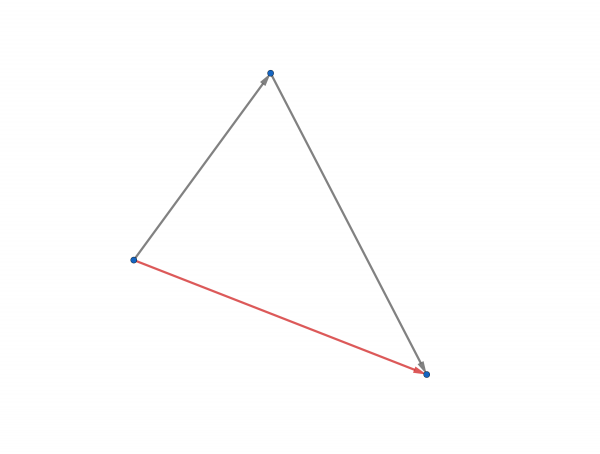Exercise. What do symmetry and reflexivity look like in the digraph?